Function Repository Resource:

# BinomialMod

Efficient computation of a binomial coefficient modulo a given number

Contributed by: Aster Ctor
 ResourceFunction["BinomialMod"][n,m,p] is equivalent to Mod[Binomial[n,m],p].

## Details and Options

ResourceFunction["BinomialMod"] uses some mathematical theory to speed up operations.
If the modulus is prime, then it uses Lucas’s theorem.
If it is square-free, then Lucas’s theorem is used on the prime factors, and the Chinese Remainder algorithm is used to form the final result.

## Examples

### Basic Examples (1)

The following formula illustrates the fundamental equivalence with Mod[Binomial[]]:

 In:=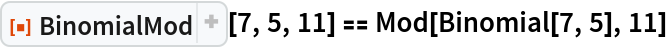Out=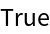### Scope (6)

#### Prime Case (3)

1299709 is a prime number:

 In:=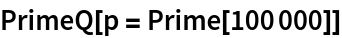Out=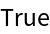Ordinary algorithms take a long time:

 In:=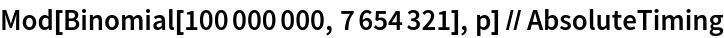Out=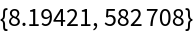The optimized algorithm is much faster:

 In:=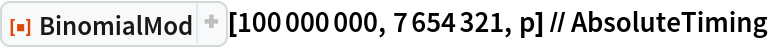Out=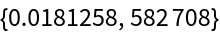#### Square-Free Case (3)

1005973 is a square-free number:

 In:=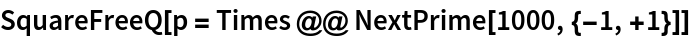Out=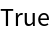Ordinary algorithms take a long time:

 In:=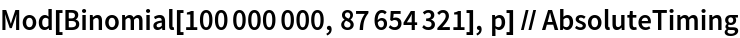Out=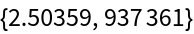The optimized algorithm is much faster:

 In:=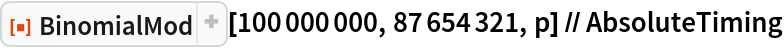Out=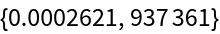### Possible Issues (2)

Handling of non-square moduli can be slow:

 In:=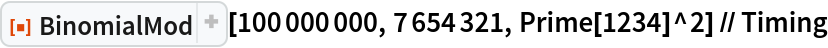Out=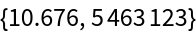In such cases the code simply uses Mod[Binomial[]]:

 In:=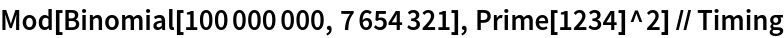Out=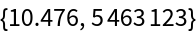## Publisher

Aster Ctor (MoeNet)

## Version History

• 1.0.0 – 06 November 2019

## Author Notes

Acceleration of non-square free numbers is possible, but I still don't fully understand the mathematical theory needed. For more information, see https://math.stackexchange.com/questions/222637/binomial-coefficient-modulo-prime-power.